# CREATE FUNCTION

## Purpose

Use this statement to create a user-defined function.

## Prerequisites

• You need either the system privilege USE ANY SCHEMA or the object privilege USAGE on the target schema, or the schema must be owned by you or one of your assigned roles.

• You need the system privilegeCREATE ANY FUNCTION, or CREATE FUNCTION if the function is created in your own schema or in that of an assigned role.
• If the option OR REPLACE is specified and the function already exists, then you also need to have the rights for DROP FUNCTION.
• To use a function, you need to have the system privilege EXECUTE ANY FUNCTION, the object privilege EXECUTE on the function or its schema, or be the current owner.

## Syntax

create_function::=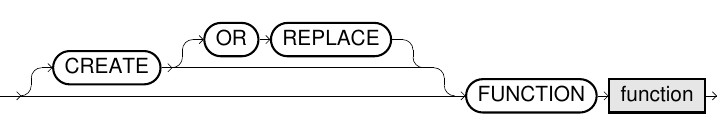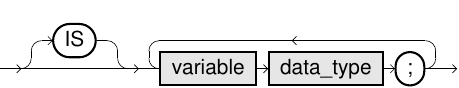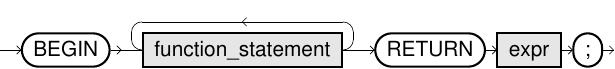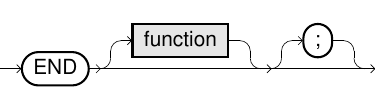function_statement::=

assignment::=if_branch::=for_loop::=for_loop_2::=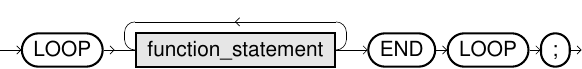while_loop::=## Usage Notes

• The option OR REPLACE can be used to replace an existing function without having to explicitly delete it with DROP FUNCTION.
• For variable declarations, the normal SQL data types are valid. Refer to Data Types for more information.
• Variable assignments cannot be performed in the variable declaration, but only in the function body.
• Any scalar SQL expressions can be used for expressions (expr). For example, all built-in and previously defined scalar functions are available. For more information, refer to the Scalar Functions section.
• Similar to normal SQL statements any number of comments can be inserted within a function definition. For more information, refer to the Comments in SQL section.
• The counter variable of the FOR statement iterates over all integers which are defined by the border parameters. The number of iterations is evaluated as a constant before entering the FOR body, and it is never re-evaluated.
• The definition text of a user-defined function can be found in the EXA_ALL_FUNCTIONS system table.
• Scalar subqueries within functions cannot be parametrized. That means they cannot contain variables or parameters.

## Examples

``CREATE OR REPLACE FUNCTION percentage (fraction DECIMAL,                                       entirety DECIMAL)   RETURN VARCHAR(10)   IS     res DECIMAL;   BEGIN     IF entirety = 0       THEN res := NULL;     ELSE       res := (100*fraction)/entirety;     END IF;     RETURN res || ' %';   END percentage;/SELECT fraction, entirety, percentage(fraction,entirety) AS PERCENTAGEFROM my_table;``

## Results

FRACTION ENTIRETY PERCENTAGE
1 2 50%
1 3 33%
3 24 12%
``-- Examples for function statements-- assignment    res := CASE WHEN input_variable < 0 THEN 0 ELSE input_variable END;``
``-- if-branch    IF input_variable = 0 THEN      res := NULL;    ELSE      res := input_variable;    END IF;``
``-- for loop    FOR cnt := 1 TO input_variable    DO        res := res*2;    END FOR;``
``-- while loop    WHILE cnt <= input_variable    DO         res := res*2;         cnt := cnt+1;    END WHILE;``